Courses

# Probability Questions with Answers Quant Notes | EduRev

## General Aptitude for GATE 2020

Created by: Wizius Careers

## Quant : Probability Questions with Answers Quant Notes | EduRev

The document Probability Questions with Answers Quant Notes | EduRev is a part of the Quant Course General Aptitude for GATE 2020.
All you need of Quant at this link: Quant

Question 1:  One ticket is selected at random from 50 tickets numbered  0, 01, 02, ……, 49.   Then,   the probability that the sum of the digits on the selected ticket is 8, given that the product of these digits is zero equals

(a) 1/14
(b) 1/7
(c) 5/14
(d) 1/50

Explanation: (a)

Here we have

Let A be the event that sum of the digits on the selected ticket is 8, then

Let B be the event that the product of the digits is zero.

B = {00, 01, 02, 03,....., 09, 10, 20, 30, 40}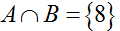∴ required probabilty = P(A/B)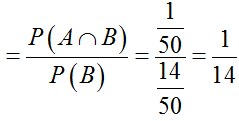Question 2: It is given that the events A and B are such that P(A) = ¼ , P(A|B) = ½ and P(B|A) = 2/3. Then P(B|A) = 2/3. Then P(B) is
(a) 1/2
(b) 1/6
(c) 1/3
(d) 2/3

Explanation: (c)

Given that P(A) = 1/4,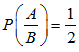and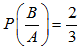we know,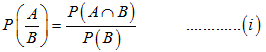and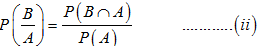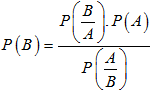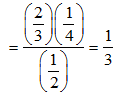Question 3: A die is thrown. Let A be the event that the number obtained is greater than 3. Let B be the event that the number obtained is less than 5. Then P (AUB) is
(a) 2/5
(b) 3/5
(c) 0
(d) 1

Explanation: (d)

Since, A {4,5,6} and B = {1,2,3,4}

∴ A∩B = {4}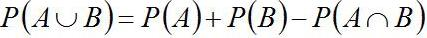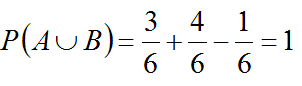Question 4: A pair of fair dice is thrown independently three times. The probability of getting a total of exactly 9 twice is

(a) 1/729
(b) 8/9
(c) 8/729
(d) 8/243

Explanation: (d)

Probability of getting a total of 9 in a single throw

4/36 = 1/9

Probability of getting a total of 9 exactly in double throw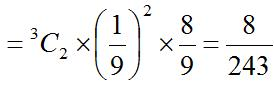Question 5: Two aeroplanes I and II bomb a target in succession. The probabilities of I and II scoring a hit correctly are 0.3 and 0.2, respectively. The second plane will bomb only if the first misses the target. The probability that the target is hit by the second plane, is
(a) 0.06
(b) 0.14
(c) 0.32
(d) 0.7

Explanation: (c)

Let the events,

A = 1st aeroplane hit the target

B =2nd aeroplane hit the target

And their corresponding probabilities are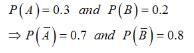Required Probability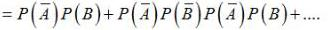= (0.7)(0.2) + (0.7)(0.8)(0.7)(0.2)+(0.7)(0.8)(0.7)(0.8)(0.7)(0.2) + ....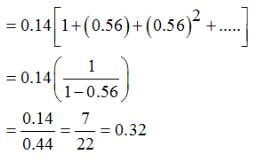## General Aptitude for GATE 2020

59 videos|47 docs|108 tests

,

,

,

,

,

,

,

,

,

,

,

,

,

,

,

,

,

,

,

,

,

;